Advertisement Remove all ads

# NCERT solutions for Class 8 Maths chapter 5 - Data Handling [Latest edition]

#### Chapters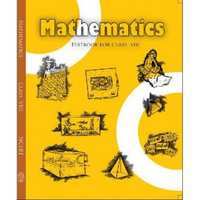Advertisement Remove all ads
Advertisement Remove all ads
Advertisement Remove all ads

## Chapter 5: Data Handling

Exercise 5.1Exercise 5.2Exercise 5.3
Advertisement Remove all ads
Exercise 5.1 [Page 76]

### NCERT solutions for Class 8 Maths Chapter 5 Data HandlingExercise 5.1 [Page 76]

Exercise 5.1 | Q 1 | Page 76

For which of these would you use a histogram to show the data?

(a) The number of letters for different areas in a postman’s bag.

(b) The height of competitors in an athletics meet.

(c) The number of cassettes produced by 5 companies.

(d) The number of passengers boarding trains from 7:00 a.m. to 7:00 p.m. at a station.

Give reasons for each.

Exercise 5.1 | Q 2 | Page 76

The shoppers who come to a departmental store are marked as: man (M), woman (W), boy (B) or girl (G). The following list gives the shoppers who came during the first hour in the morning

W W W G B W W M G G M M W W W W G B M W B G G M W W M M W W W M W B W G M W W W W G W M M W W M W G W M G W M M B G G W

Make a frequency distribution table using tally marks. Draw a bar graph to illustrate it.

Exercise 5.1 | Q 3 | Page 76

The weekly wages (in Rs) of 30 workers in a factory are.

830, 835, 890, 810, 835, 836, 869, 845, 898, 890, 820, 860, 832, 833, 855, 845, 804, 808, 812, 840, 885, 835, 835, 836, 878, 840, 868, 890, 806, 840

Using tally marks make a frequency table with intervals as 800 − 810, 810 − 820 and so on.

Exercise 5.1 | Q 4 | Page 76

Draw a histogram for the frequency table made for the data in Question 3 and answer the following questions.

(1) Which group has the maximum number of workers?

(2) How many workers earn Rs 850 and more?

(3) How many workers earn less than Rs 850?

Exercise 5.1 | Q 5 | Page 76

The number of hours for which students of a particular class watched television during holidays is shown through the given graph.

Answer the following

1) For how many hours did the maximum number of students watch TV?

2) How many students watched TV for less than 4 hours?

3) How many students spent more than 5 hours in watching TV?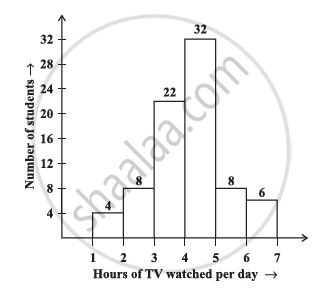Exercise 5.2 [Page 82]

### NCERT solutions for Class 8 Maths Chapter 5 Data HandlingExercise 5.2 [Page 82]

Exercise 5.2 | Q 1 | Page 82

A survey was made to find the type of music that a certain group of young people liked in a city. Adjoining pie chart shows the findings of this survey.

From this pie chart answer the following −

1) If 20 people liked classical music, how many young people were surveyed?

2) Which type of music is liked by the maximum number of people?

3) If a cassette company were to make 1000 CD’s, how many of each type would they make?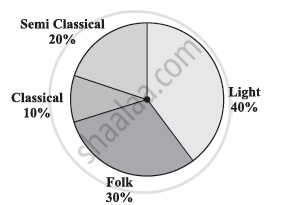Exercise 5.2 | Q 2 | Page 82

A group of 360 people were asked to vote for their favourite season from the three seasons rainy, winter and summer.

(1) Which season got the most votes?

(2) Find the central angle of each sector.

(3) Draw a pie chart to show this information.

 Season No. of votes Summer 90 Rainy 120 Winter 150
Exercise 5.2 | Q 3 | Page 82

Draw a pie chart showing the following information. The table shows the colours preferred by a group of people.

 Colours Number of people Blue 18 Green 9 Red 6 Yellow 3 Total 36
Exercise 5.2 | Q 4 | Page 82

The adjoining pie chart gives the marks scored in an examination by a student in Hindi, English, Mathematics, Social Science and Science. If the total marks obtained by the students were 540, answer the following questions.

1) In which subject did the student score 105 marks?

(Hint: For 540 marks, the central angle = 360°. So, for 105 marks, what is the central angle?)

2) How many more marks were obtained by the student in Mathematics than in Hindi?

3) Examine whether the sum of the marks obtained in Social Science and Mathematics is more than that in Science and Hindi.

(Hint: Just study the central angles)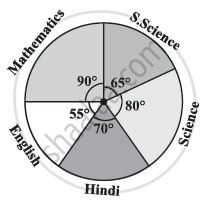Exercise 5.2 | Q 5 | Page 82

The number of students in a hostel, speaking different languages is given below. Display the data in a pie chart.

 Language Hindi English Marathi Tamil Bengali Total Number of students 40 12 9 7 4 72
Exercise 5.3 [Page 87]

### NCERT solutions for Class 8 Maths Chapter 5 Data HandlingExercise 5.3 [Page 87]

Exercise 5.3 | Q 1.1 | Page 87

List the outcomes you can see in these experiments.

Spinning a wheel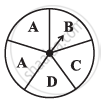Exercise 5.3 | Q 1.2 | Page 87

List the outcomes you can see in these experiments.

Tossing two coins together

Exercise 5.3 | Q 2.1 | Page 87

When a die is thrown, list the outcomes of an event of getting

a) a prime number

b) not a prime number

Exercise 5.3 | Q 2.2 | Page 87

When a die is thrown, list the outcomes of an event of getting

a) a number greater than 5

b) a number not greater than 5

Exercise 5.3 | Q 3.1 | Page 87

Find the Probability of the pointer stopping on D in (Question 1 − (a))?

Exercise 5.3 | Q 3.2 | Page 87

Find the Probability of getting an ace from a well shuffled deck of 52 playing cards?

Exercise 5.3 | Q 3.3 | Page 87

Find the Probability of getting a red apple. (See figure below)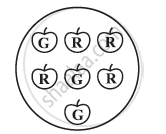Exercise 5.3 | Q 4 | Page 87

Numbers 1 to 10 are written on ten separate slips (one number on one slip), kept in a box and mixed well. One slip is chosen from the box without looking into it. What is the probability of.

(1) getting a number 6?

(2) getting a number less than 6?

(3) getting a number greater than 6?

(4) getting a 1-digit number?

Exercise 5.3 | Q 5 | Page 87

If you have a spinning wheel with 3 green sectors, 1 blue sector and 1 red sector, what is the probability of getting a green sector? What is the probability of getting a non blue sector?

Exercise 5.3 | Q 6 | Page 87

Find the probabilities of the events given in Question 2.

## Chapter 5: Data Handling

Exercise 5.1Exercise 5.2Exercise 5.3## NCERT solutions for Class 8 Maths chapter 5 - Data Handling

NCERT solutions for Class 8 Maths chapter 5 (Data Handling) include all questions with solution and detail explanation. This will clear students doubts about any question and improve application skills while preparing for board exams. The detailed, step-by-step solutions will help you understand the concepts better and clear your confusions, if any. Shaalaa.com has the CBSE Class 8 Maths solutions in a manner that help students grasp basic concepts better and faster.

Further, we at Shaalaa.com provide such solutions so that students can prepare for written exams. NCERT textbook solutions can be a core help for self-study and acts as a perfect self-help guidance for students.

Concepts covered in Class 8 Maths chapter 5 Data Handling are Graphical Representation of Data as Histograms, Organisation of Data, Concept of Data Handling, Drawing a Bar Graph, Drawing a Double Bar Graph, Interpretation of a Double Bar Graph, Frequency Distribution Table, Concept of Pie Graph (Or a Circle-graph), Interpretation of Pie Diagram, Chance and Probability - Chance, Interpretation of a Pictograph, Interpretation of Bar Graphs, Basic Ideas of Probability.

Using NCERT Class 8 solutions Data Handling exercise by students are an easy way to prepare for the exams, as they involve solutions arranged chapter-wise also page wise. The questions involved in NCERT Solutions are important questions that can be asked in the final exam. Maximum students of CBSE Class 8 prefer NCERT Textbook Solutions to score more in exam.

Get the free view of chapter 5 Data Handling Class 8 extra questions for Class 8 Maths and can use Shaalaa.com to keep it handy for your exam preparation

Advertisement Remove all ads
Share
Notifications

View all notifications

Forgot password?